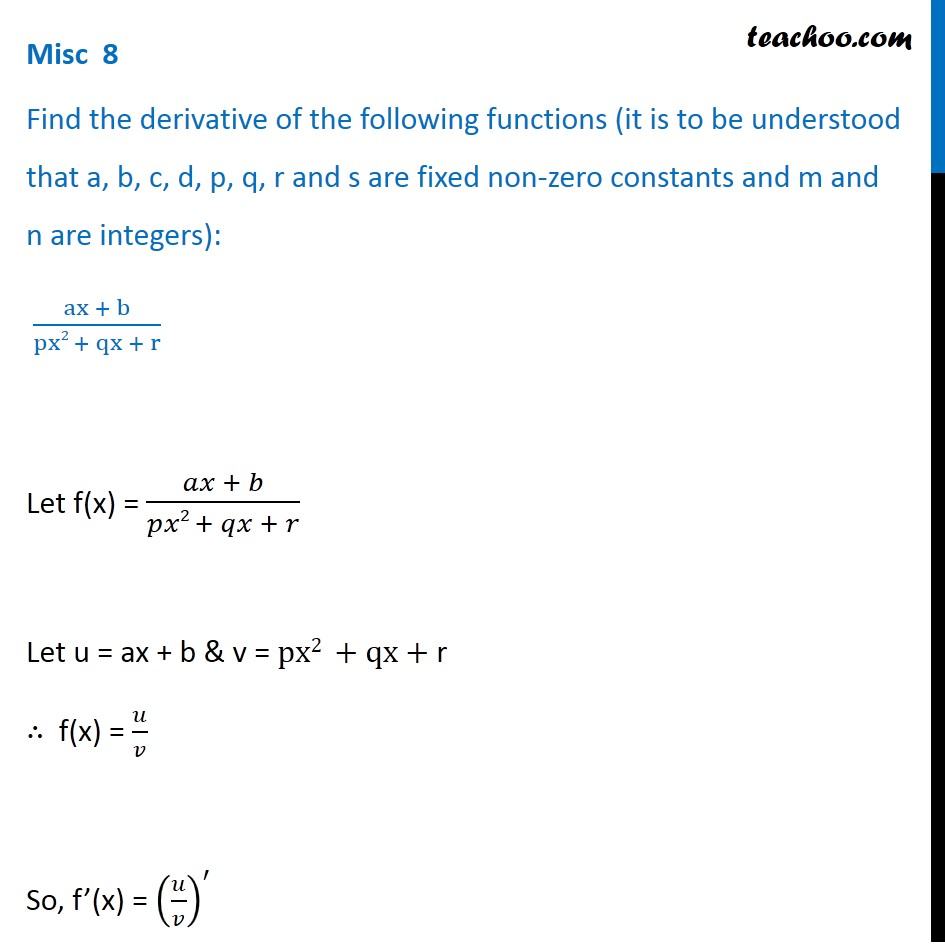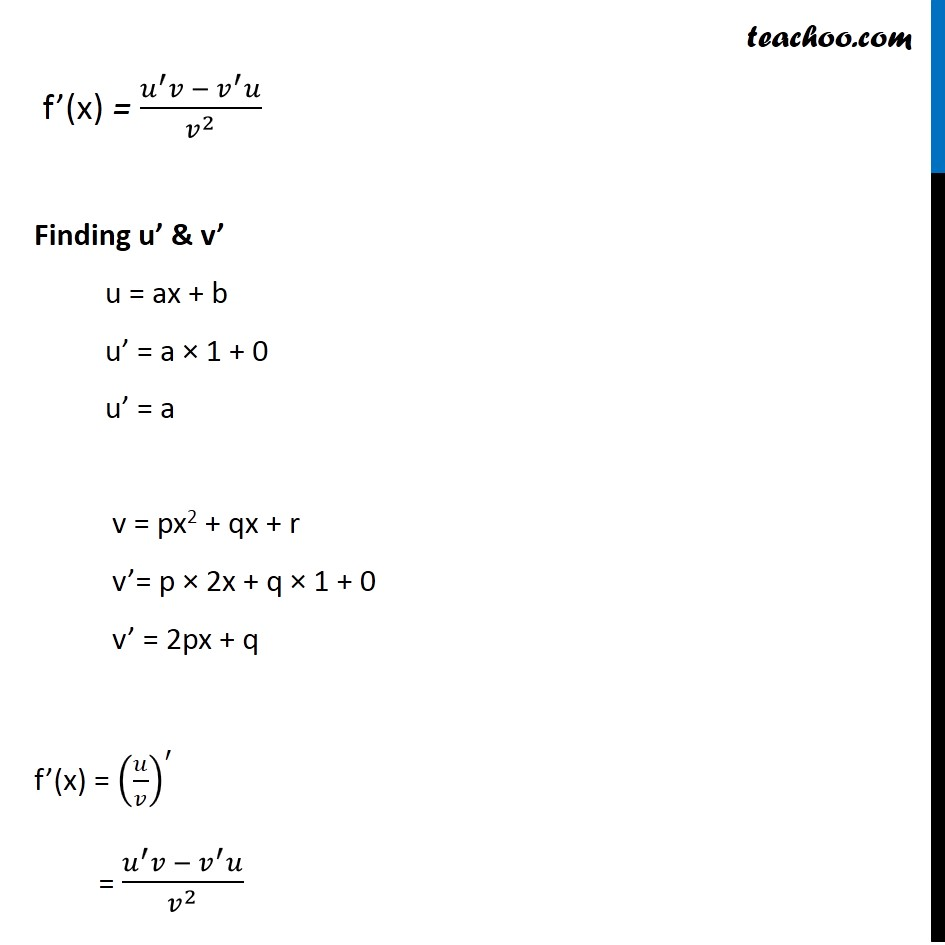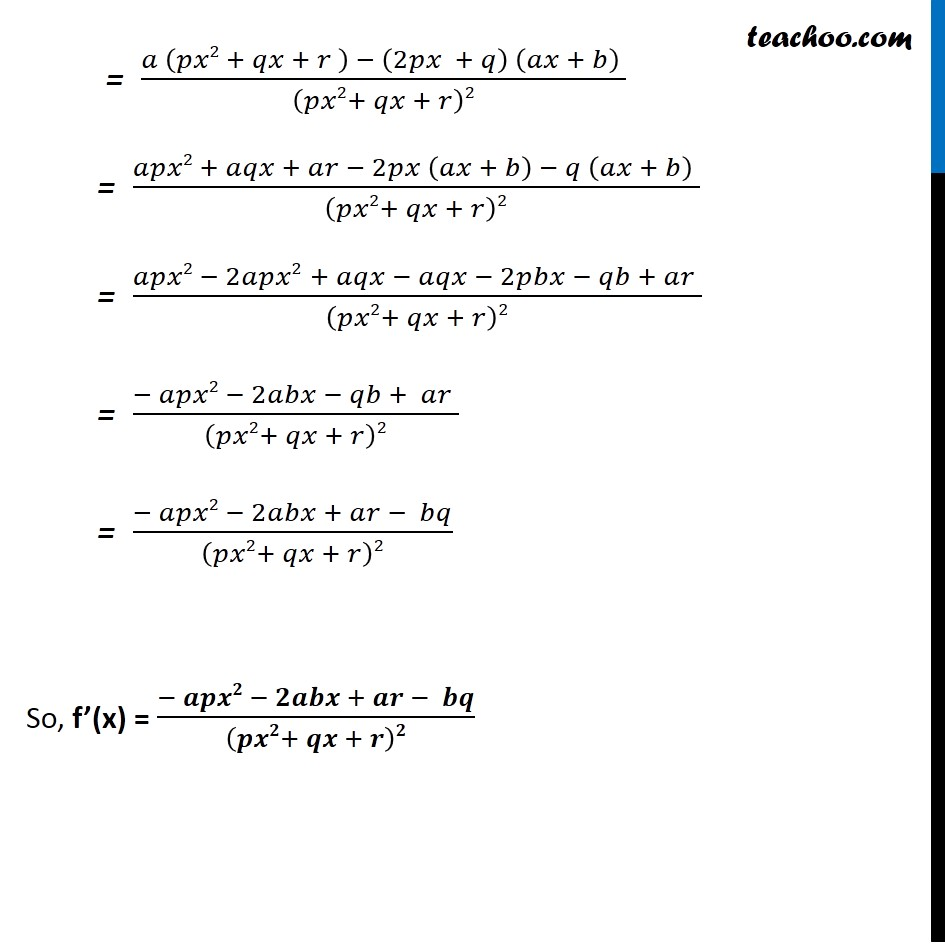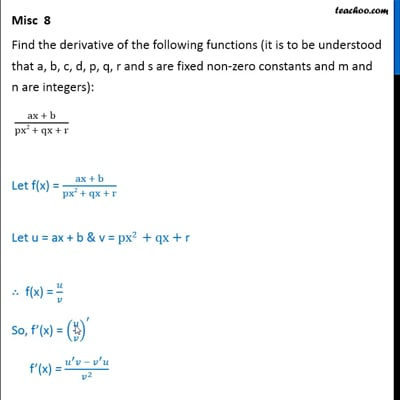Miscellaneous

Chapter 13 Class 11 Limits and Derivatives
Serial order wiseThis video is only available for Teachoo black users

Introducing your new favourite teacher - Teachoo Black, at only ₹83 per month

### Transcript

Misc 8 Find the derivative of the following functions (it is to be understood that a, b, c, d, p, q, r and s are fixed non-zero constants and m and n are integers): (ax + b)/(px2 + qx + r) Let f(x) = (𝑎𝑥 + 𝑏)/(𝑝𝑥2 + 𝑞𝑥 + 𝑟) Let u = ax + b & v = px2 +qx+ r ∴ f(x) = 𝑢/𝑣 So, f’(x) = (𝑢/𝑣)^′ f’(x) = (𝑢^′ 𝑣 − 𝑣^′ 𝑢)/𝑣^2 Finding u’ & v’ u = ax + b u’ = a × 1 + 0 u’ = a v = px2 + qx + r v’= p × 2x + q × 1 + 0 v’ = 2px + q f’(x) = (𝑢/𝑣)^′ = (𝑢^′ 𝑣 −〖 𝑣〗^′ 𝑢)/𝑣^2 = (𝑎 (𝑝𝑥2 + 𝑞𝑥 + 𝑟 ) − (2𝑝𝑥 + 𝑞) (𝑎𝑥 + 𝑏) )/(𝑝𝑥2+ 𝑞𝑥 + 𝑟)2 = (𝑎𝑝𝑥2 + 𝑎𝑞𝑥 + 𝑎𝑟 − 2𝑝𝑥 (𝑎𝑥 + 𝑏) − 𝑞 (𝑎𝑥 + 𝑏) )/(𝑝𝑥2+ 𝑞𝑥 + 𝑟)2 = (𝑎𝑝𝑥2 − 2𝑎𝑝𝑥2 + 𝑎𝑞𝑥 − 𝑎𝑞𝑥 − 2𝑝𝑏𝑥 − 𝑞𝑏 + 𝑎𝑟 )/(𝑝𝑥2+ 𝑞𝑥 + 𝑟)2 = (− 𝑎𝑝𝑥2 − 2𝑎𝑏𝑥 − 𝑞𝑏 + 𝑎𝑟 )/(𝑝𝑥2+ 𝑞𝑥 + 𝑟)2 = (− 𝑎𝑝𝑥2 − 2𝑎𝑏𝑥 + 𝑎𝑟 − 𝑏𝑞)/(𝑝𝑥2+ 𝑞𝑥 + 𝑟)2 So, f’(x) = (− 𝒂𝒑𝒙𝟐 − 𝟐𝒂𝒃𝒙 + 𝒂𝒓 − 𝒃𝒒)/(𝒑𝒙𝟐+ 𝒒𝒙 + 𝒓)𝟐